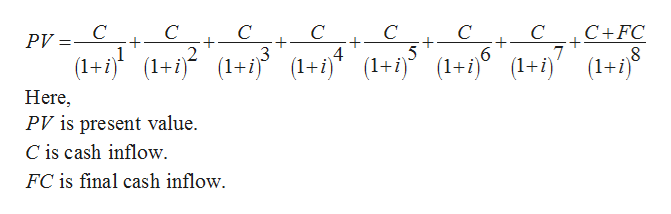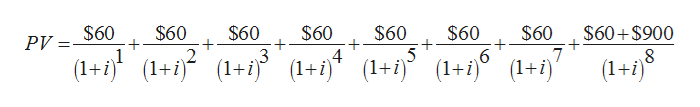# You buy a mutual fund for \$1,000. It annually distributes \$60 for eight years, after which you sell the shares for \$900. What is the annualized return on your investment?

Question
6 views

You buy a mutual fund for \$1,000. It annually distributes \$60 for eight years, after which you sell the shares for \$900. What is the annualized return on your investment?

check_circle

Step 1

Expected rate of return can be described as the total profit or loss on a particular investment over a set period of time. Expected rate of return is generally stated as a percentage in relation to primary cost of investment.

It is given that,

Initial cash outflow is \$1,000.

Annual cash inflow is \$60.

Cash inflow at the end of year 8 is \$900.

Step 2

The formula to calculate present value will be helpful to calculate the expected interest rate.

The below expression can be used to calculate expected interest rate:help_outlineImage TranscriptioncloseC+FC C C + C + C + PV + 7 (1+((1+ Here PV is present value. C is cash inflow FC is final cash inflow (1+ij (1+j6 (1+ 5 ( 2 3 8 1+i)(1+ (1+) (1 i) fullscreen
Step 3

Substitute \$60 for C and \$900 for FC...help_outlineImage Transcriptionclose\$60 \$60 2 \$60 \$60 \$60 + 4 \$60 6 \$60 \$60+\$900 7 (1+) PV 5 (+(1+(1+(1+1 (1+j (1+j (L+i) 3 (1+i) (1+f)(1+(+i) (1+i) fullscreen

### Want to see the full answer?

See Solution

#### Want to see this answer and more?

Solutions are written by subject experts who are available 24/7. Questions are typically answered within 1 hour.*

See Solution
*Response times may vary by subject and question.
Tagged in